# US7401001B2 - Position determining - Google Patents

## Info

Publication number
US7401001B2
US7401001B2 US10/579,418 US57941804A US7401001B2 US 7401001 B2 US7401001 B2 US 7401001B2 US 57941804 A US57941804 A US 57941804A US 7401001 B2 US7401001 B2 US 7401001B2
Authority
US
United States
Prior art keywords
component
cosine
sine
value
amplitude
Prior art date
Legal status (The legal status is an assumption and is not a legal conclusion. Google has not performed a legal analysis and makes no representation as to the accuracy of the status listed.)
Expired - Fee Related
Application number
US10/579,418
Other versions
US20070093980A1 (en
Inventor
Aalbert Stek
Anthonius Petrus Gerardus Emanuel Janssen
Current Assignee (The listed assignees may be inaccurate. Google has not performed a legal analysis and makes no representation or warranty as to the accuracy of the list.)
Koninklijke Philips NV
Original Assignee
Koninklijke Philips NV
Priority date (The priority date is an assumption and is not a legal conclusion. Google has not performed a legal analysis and makes no representation as to the accuracy of the date listed.)
Filing date
Publication date
Priority to EP03104243.5 priority Critical
Priority to EP03104243 priority
Application filed by Koninklijke Philips NV filed Critical Koninklijke Philips NV
Priority to PCT/IB2004/052410 priority patent/WO2005050140A1/en
Assigned to KONINKLIJKE PHILIPS ELECTRONICS, N.V. reassignment KONINKLIJKE PHILIPS ELECTRONICS, N.V. ASSIGNMENT OF ASSIGNORS INTEREST (SEE DOCUMENT FOR DETAILS). Assignors: JANSSEN, ANTONIUS PETRUS GERARDUS EMANUEL, STEK, AALBERT
Publication of US20070093980A1 publication Critical patent/US20070093980A1/en
Publication of US7401001B2 publication Critical patent/US7401001B2/en
Application granted granted Critical
Expired - Fee Related legal-status Critical Current
Anticipated expiration legal-status Critical

• 230000000694 effects Effects 0.000 description 1

## Images

•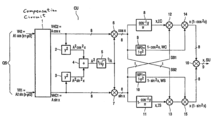•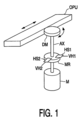•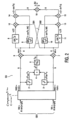•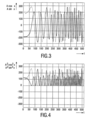•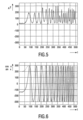•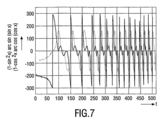•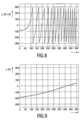## Classifications

• GPHYSICS
• G01MEASURING; TESTING
• G01DMEASURING NOT SPECIALLY ADAPTED FOR A SPECIFIC VARIABLE; ARRANGEMENTS FOR MEASURING TWO OR MORE VARIABLES NOT COVERED IN A SINGLE OTHER SUBCLASS; TARIFF METERING APPARATUS; MEASURING OR TESTING NOT OTHERWISE PROVIDED FOR
• G01D5/00Mechanical means for transferring the output of a sensing member; Means for converting the output of a sensing member to another variable where the form or nature of the sensing member does not constrain the means for converting; Transducers not specially adapted for a specific variable
• G01D5/12Mechanical means for transferring the output of a sensing member; Means for converting the output of a sensing member to another variable where the form or nature of the sensing member does not constrain the means for converting; Transducers not specially adapted for a specific variable using electric or magnetic means
• G01D5/244Mechanical means for transferring the output of a sensing member; Means for converting the output of a sensing member to another variable where the form or nature of the sensing member does not constrain the means for converting; Transducers not specially adapted for a specific variable using electric or magnetic means influencing characteristics of pulses or pulse trains; generating pulses or pulse trains
• G01D5/24471Error correction
• G01D5/2448Correction of gain, threshold, offset or phase control

## Abstract

A position determining system for determining a position of a rotor of a rotating motor has sensors that are coupled to the rotor. The sensors generate, in response to a rotation of the rotor, a quadrature signal that has sine and cosine components. The position determining system calculates a sum (A2) of a squared value of the sine component (A2sin2x) and a squared value of the cosine component (A2cos2x). An amplitude correction factor (A) is calculated as the squared root of the sum (A2). An amplitude corrected sine component (sin(x)) is obtained by dividing the sine component (Asin(x)) by the amplitude correction factor (A). An amplitude corrected cosine component (cos(x)) is obtained by dividing the cosine component (Acos(x)) by the amplitude correction factor (A).

## Description

The invention relates to a position determining system for determining a position of a rotor of a rotating motor, a position determining method, and an optical or magnetic drive comprising such a position determining system.

U.S. Pat. No. 5,677,686 discloses an absolute position detection apparatus which comprises sine and cosine wave generators for generating one or more sets of sine and cosine waves within a cycle. An analog to digital converters converts the incoming sine and cosine waves generated by the sine and cosine wave generators into digital values. An arithmetic unit calculates a compensation for offset, amplitude and phase errors on the basis of the digital values from the analog to digital converters. The digital values supplied by the analog to digital converters include offset errors, a too large or too small amplitude, and a shift, between the two phases. U.S. Pat. No. 5,677,686 discloses a static and dynamic compensation of these errors.

In the sixth embodiment of this prior art, from the offset compensated data sin(AOF) and cos(AOF) the squared value R=sin2(AOF)+cos2(AOF) is determined. This data is sampled during operation to provide a maximum value or an average value of a given sampling count. The squared value at 90° is compared with the squared value at 270° and the offset compensation value is changed according to a difference between these squared values. For example, if the squared value at 90° has a smaller value than the squared value at 270°, the offset compensation value is increased until these squared values are equal.

In the seventh embodiment of this prior art, an amplitude compensation is disclosed which uses the squared values. The amplitude compensation value is the square root of a reference value divided by the equal squared values. The fourth embodiment of this prior art discloses that the sine and cosine are amplitude compensated by multiplying the original sine and cosine with a reference amplitude divided by the amplitude compensation value. Thus, the sine and cosine are amplitude compensated by multiplying the original sine and cosine with the square root of the squared value.

A drawback of the prior art is that the amplitude offset compensation drifts away when the motor is not rotating.

It is an object of the invention to provide a position determining system which is able to perform amplitude offset compensation even when the motor is not rotating.

A first aspect of the invention provides a position determining system for determining a position of a rotor of a rotating motor, said system comprises sensing means coupled to the rotor for generating in response to a rotation of the rotor a quadrature signal comprising a sine component and a cosine component, and calculating means for calculating (i) a sum of a squared value of the sine component and a squared value of the cosine component, (ii) an amplitude correction factor as the squared root of the sum, and (iii) an amplitude corrected sine component as the sine component divided by the amplitude correction factor and an amplitude corrected cosine component as the cosine component divided by the amplitude correction factor.

A second aspect of the invention provides a position determining method for determining a position of a rotor of a rotating motor, said method comprising generating in response to a rotation of the rotor a quadrature signal comprising a sine component and a cosine component, and calculating (i) a sum of a squared value of the sine component and a squared value of the cosine component, (ii) an amplitude correction factor as the squared root of the sum, and (iii) an amplitude corrected sine component as the sine component divided by the amplitude correction factor and an amplitude corrected cosine component as the cosine component divided by the amplitude correction factor.

A third aspect of the invention provides an optical or magnetic drive comprising a pick-up unit for reading and/or writing information from/to an optical or magnetic medium, a rotating motor with rotor, a gearbox for converting a rotating movement of the rotor into a linear movement of optical pick-up unit, and a position determining system for determining a position of the rotor, said system comprising sensing means coupled to the rotor for generating in response to a rotation of the rotor a quadrature signal comprising a sine component and a cosine component, and calculating means for calculating (i) a sum of a squared value of the sine component and a squared value of the cosine component, (ii) an amplitude correction factor as the squared root of the sum, and (iii) an amplitude corrected sine component as the sine component divided by the amplitude correction factor and an amplitude corrected cosine component as the cosine component divided by the amplitude correction factor.

The position determining system in accordance with the first aspect of the invention determines a position of a rotor of a rotating motor. The position determining system generates, in response to a rotation of the rotor, a quadrature signal which comprises a sine component and a cosine component, in the same manner as in the prior art. As long as the motor is rotating, the sine and cosine components are sine wave shaped signals. When the motor is not rotating the sine and cosine components are DC levels. The position determining system calculates an amplitude correction factor as the squared root of the sum of a squared value of the sine component and a squared value of the cosine component. The amplitude corrected sine component is the sine component divided by the amplitude correction factor, and the amplitude corrected cosine component is the cosine component divided by the amplitude correction factor.

Thus for each set of values of the sine component and the cosine component the squared root is determined. The amplitude corrected sine and cosine components are obtained by dividing the sine and cosine components by the squared root. Consequently, the amplitude correction is also operative if the motor does not rotate. In contrast, the prior art amplitude compensation has to determine minimum and maximum values to be able to perform the amplitude compensation. This is only possible as long as the motor is rotating. If the motor is not rotating, the sine and cosine components are DC levels which do not have different maximum and a minimum values. Therefore, the prior art is unable to compensate for drift of the DC-levels. This has the disadvantage that during periods in time the motor is not rotating, the position information is drifting away which will take extra time to catch the correct position when the motor starts rotating.

The amplitude correction in accordance with the invention is based on the insight that at every phase of the quadrature signal holds:
A 2 sin2(x)+A 2 cos2(x)=A 2 wherein A is the amplitude.

Thus, at every phase of the rotor, the dynamic amplitude A is determined. The amplitude corrected sine and cosine components are obtained by dividing the original sine and cosine components by this dynamic amplitude.

A further advantage of the amplitude correction in accordance with the invention is that it is instantaneous, while in the prior art cited, the compensation value is iteratively increased until these squared values are equal.

It has to be noted that in the amplitude correction in accordance with the invention the original sine and cosine components are divided by a factor proportional with the squared root of the sum of the squared values, while in the prior art cited this division is a multiplication.

In one embodiment, the position is determined by calculating: the inverse sine (by applying the arcsine function) of the amplitude corrected sine component to obtain the amplitude of the sine component, the inverse cosine (by applying the arccosine function) of the amplitude corrected cosine component to obtain the amplitude of the cosine component, and summing these two amplitude values.

In another embodiment, before the sum is calculated, first, the amplitudes of the cosine and sine component are weighted. The weighting factors or functions are selected to favor the cosine and sine component around their zero crossings. Thus, the weighting factor for the cosine or sine component has value around the zero crossings of the cosine or sine component which is larger than its value around the peaks of the cosine or sine component. For example, the weighting function is 1-cos2x or 1-sin2x for the cosine or sine component, respectively. This weighting has the advantage that the parts of the cosine or sine component where the sensitivity to the position variation is largest are favored. Thus, the weighting function suppresses the peaks of the cosine and sine component which are less sensitive to the position variation and do not suppress the steep slopes around the zero crossings.

These and other aspects of the invention are apparent from and will be elucidated with reference to the embodiments described hereinafter.

In the drawings:

FIG. 1 shows a mechanism which converts a rotating movement into a linear movement and which comprises sensors for supplying position information,

FIG. 2 shows a block diagram of a position determining system which determines a position indication from the position information,

FIG. 3 shows statically corrected position information sine and cosine signals,

FIG. 4 shows squared statically corrected position information signals,

FIG. 5 shows the dynamic gain correction factor obtained by summing the squared statically corrected position information signals,

FIG. 6 shows the dynamically normalized sine and cosine signals,

FIG. 7 shows the weighted phases of the sine and cosine signals,

FIG. 8 shows the phase derived from the weighted phases, and

FIG. 9 shows the extended phase which is the position indication indicative for the position of the motor or the element which is linearly moved.

FIG. 1 shows a mechanism which converts a rotating movement into a linear movement and which comprises sensors for supplying position information. The motor M has a rotor which rotates the shaft AX when the motor is energized. The rotor is inside the motor housing and thus is not shown. Usually, in an optical drive, the shaft AX is connected to a gearbox which decreases the rotating speed of the rotor to a desired rotating speed of an outgoing axis. The gearbox may further comprise a construction for converting the rotating speed of the outgoing axis to a linear movement of an optical pickup unit. In FIG. 1, a simplified gearbox is shown. This gearbox comprises a disc shaped member DM attached to the shaft AX. A side wall of the disc shaped member DM is pushed against a rod shaped element which is or is part of the optical unit OPU. The practical implementation of the gearbox is not relevant to the invention and may have any suitable construction which is able to convert the rotating movement of the shaft AX connected to the rotor into a linear movement of the optical unit OPU. Although is referred to the optical unit which is used in an optical disc drive to cooperate which an optical disc, this unit may also be a magnetical unit cooperating with a magnetical storage medium.

A magnetized ring MR is attached to the shaft AX to be able to determine the position of the shaft AX and thus the position of the unit OPU. Usually, this ring MR is periodically magnetized, for example, nine magnetic pole pairs may be present. Two Hall sensors HS1 and HS2 are arranged with respect to the magnetized ring MR to sense its flux. When the shaft AX of the rotor is rotating, the magnetic ring MR rotates and the Hall elements HS1 and HS2 sense the varying magnetic field which results in the sinusoidal signals VH1 and VH2, respectively. By positioning the Hall sensors HS1, HS2 under a 90° angle, the phase difference of the two signals VH1 and VH2 is 90° and a quadrature signal QS (see FIG. 2) is obtained which comprises a sine and a cosine component. The signal VH1 is the sine component and the signal VH2 is the cosine component. The position determining system shown in FIG. 2 converts this quadrature signal QS into a phase signal x,SU indicating a rotational position of the shaft AX or a linear position of the unit OPU. The phase signal x,SU is also referred to as the position signal or the position.

Instead of the Hall sensors HS1, HS2, the varying magnetic field may be sensed by other sensors which are sensitive to a magnetic field. It is also possible to sense the position by using a varying electrical field or a varying amount of light. The invention is relevant to determine a position in a system wherein a quadrature signal QS is representative for a rotating movement.

A main problem in converting the quadrature signal QS into a position signal x,SU is that, usually, the sine component VH1 and the cosine component VH2 have amplitude errors and DC-offset errors. For example, the signals VH1 and VH2 supplied by the Hall sensors HS1 and HS2 may be dependent on the temperature, the distance between the magnetized ring MR and the Hall sensors HS1 and HS2 and the strength of the magnetic field of the magnetized areas of the ring MR. As known, the signals VH1 and VH2 can be corrected for static errors. The corrected signals are indicated by VHC1 and VHC2 in FIG. 2. The corrected signals VHC1 and VHC2 are statically corrected for the average DC-offset and the average amplitude. Usually, this static correction is the only signal conditioning applied to the signal before it enters the conventional quadrature decoding algorithm. The rotation dependent variations of the signal are not cancelled. The position determining system and method in accordance with the invention provides a more advanced pre-conditioning of the signals VH1 and VH2 and, consequently, a more accurate and robust position signal x,SU results.

FIG. 2 shows a block diagram of a position determining system which determines a position indication x,SU from the position information. The static compensation circuit 1 receives the quadrature signal QS which comprises the sine component VH1 which is A2sin(x−φ/2) and the cosine component VH2 which is A1cos(x+φ/2) from the Hall sensors HS1 and HS2, respectively. The static compensation circuit 1 supplies the statically corrected position information VHC1 which is Asin(x) and VHC2 which is Acos(x). An example of these signals VHC1 and VHC2 is shown in FIG. 3. In the embodiment shown in FIG. 2, the signals VHC1 and VHC2 are 8 bit digital words as indicated by the 8 at the respective lines.

The blocks, in which a function is shown, perform these functions on their respective input signals to obtain the respective output signals. The blocks may represent hardware circuits or steps performed by a processing circuit such as a microcomputer.

The block 2 receives the signal VHC2=Acos(x) as the input signal and supplies the squared input signal A2cos2x as the output signal. The block 3 receives the signal VHC1=Asin(x) as the input signal and supplies the squared input signal A2sin2x as the output signal. The squared signals A2cos2x and A2sin2x of the blocks 2 and 3 are shown in FIG. 4 for the signals VHC1 and VHC2 shown by way of example in FIG. 3. The block 4 sums the output signals A2cos2x and A2sin2x of the blocks 2 and 3 to obtain the dynamic gain correction value A2 which is representative for the actual amplitude A at each phase x of the quadrature signal QS. In the static gain calibration performed in the static compensation circuit 1, care is taken that the amplitude A of the statically corrected sine component VHC1 and the statically corrected cosine component VHC2 are identical. The gain correction value A2 is shown in FIG. 5 for the signals VHC1 and VHC2 shown by way of example in FIG. 3.

The block 5 calculates the inverse of the square root of the value A2 to obtain a correction factor 1/A. The multiplier 6 multiplies the signal VHC2 with the correction factor 1/A to obtain the dynamically normalized signal cos(x). The multiplier 7 multiplies the signal VHC1 with the correction factor 1/A to obtain the dynamically normalized signal sin(x). The normalized signals sin(x) and cos(x) are shown in FIG. 6 for the signals VHC1 and VHC2 shown by way of example in FIG. 3. These normalized signals sin(x) and cos(x) need not have unity peak amplitude, they may have any desired fixed peak amplitude.

The actual amplitude of the signals cos(x) and sin(x) can now be converted into the corresponding phase x,SU. First, the sin(x) signal is converted into the value x,IS with the arcsin(x) function of block 11 which calculates the value x,IS as arcsin(sin(x)). And the cos(x) signal is converted into the value x,IC with the arccos(x) function of block 10 which calculates the value x,IC as arccos(cosx). The converted values x,IS and x,IC are summed in the block 16 to obtain the phase x,SU.

However, in an improved embodiment, the blocks 9 and 14 are added to weight the cos(x) signal, and the blocks 10 and 15 are added to weight the sin(x) signal. The weighting is based on the recognition that the sensitivity to a position variation is largest around the zero crossings of the signals and lowest around the peaks of the signals. Therefore, the weighting functions are selected to favor the signals around their zero crossings. The block 9 calculates the value 1-cos2(x) which is multiplied with the signal x,IC in the multiplier 14 to obtain the signal x(1-cos2(x)). The block 10 calculates the value 1-sin2(x) which is multiplied with the signal x,IS in the multiplier 15 to obtain the signal x(1-sin2(x)). The signals (1-cos2(x))arccos(cos(x)) and (1-sin2(x))arcsin(sin(x)) are shown in FIG. 7 for the signals VHC1 and VHC2 shown by way of example in FIG. 3.

The block 16 adds these signals to obtain x−xcos2(x)+x−xsin2(x)=2x−x=x indicated by x,SU (see FIG. 8) to make clear that this is the phase signal at the output of the position determining system or method.

The multipliers 12 and 13 are used to correct for a 2π wrap around as will be elucidated with respect to FIGS. 8 and 9. The multiplier 12 receives the 8 bit signal x,IC and a sign bit SB1 to supply an output signal to the multiplier 14. The multiplier 13 receives the 8 bit signal x,IS and a sign bit SB2 to supply an output signal to the multiplier 15.

FIG. 3 shows statically corrected position information sine and cosine signals. The statically corrected position information VHC1 represents the sine signal Asin(x), the statically corrected position information VHC2 represents the cosine signal Acos(x).

FIG. 4 shows the squared statically corrected position information signals A2cos2x and A2sin2x.

FIG. 5 shows the dynamic gain correction factor A2 obtained by summing the squared statically corrected position information signals.

FIG. 6 shows the dynamically normalized sine signal sin(x) and cosine signal cos(x). As is clear from FIG. 6 the Asin(x) signal and the Acos(x) signal shown in FIG. 3 are now divided by the factor A for every phase. The resultant signals sin(x) and cos(x) have the desired normalized amplitude. If these resultant signals are processed by an inverse sine function arcsin(x) and an inverse cosine function arccos(x) the corresponding phases are retrieved. The sum of these retrieved phases is the phase of the quadrature signal and thus represents the position of the shaft AX or the rotor of the motor M.

FIG. 7 shows the weighted phases of the sine and cosine signals. The weighting of the signals sin(x) and cos(x) has the effect that the parts of these signals around their zero crossings are more decisive than the parts around their peak values. This has the advantage that the parts which are most sensitive to position variations have the largest influence.

FIG. 8 shows the phase x,SU derived from the weighted phases if the multipliers 12 and 13 are not present. Two peaks with the same polarity indicate a phase change of 2π. By detecting the 2π wrap-around, the phase characteristic can be extended by subtracting 2π at the wrap-around points to obtain the smooth position signal shown in FIG. 9. The sign reversal is obtained by the sign bits SB1 and SB2 supplied to the multipliers 12 and 13, respectively.

FIG. 9 shows the extended phase x,SU when the multipliers 12 and 13 are present. This extended phase x,SU is the position indication indicative for the rotational position of the shaft AX or the linear position of the element OPU which is linearly moved.

It should be noted that the above-mentioned embodiments illustrate rather than limit the invention, and that those skilled in the art will be able to design many alternative embodiments without departing from the scope of the appended claims.

For example, the circuit shown in FIG. 2 may comprise dedicated hardware circuits performing the actions or operations described. Preferably, the signals VH1, VH2 supplied by the Hall sensors HS1, HS2 are digitized by analog the digital converters to enable digital signal processing. The number of bits of the digital words representing the signals mentioned may be selected to obtain a desired accuracy. The algorithm may also be performed by a suitably programmed computer or digital processor unit. Although preferably the operations are performed in the digital domain it is as well possible to perform the operations in the analog domain.

The extended phase x,SU which is the position indication may be used in a control loop which further receives a set point. The difference between the set point and the position indication is used to generate a control signal for the motor.

In the claims, any reference signs placed between parentheses shall not be construed as limiting the claim. Use of the verb “comprise” and its conjugations does not exclude the presence of elements or steps other than those stated in a claim. The article “a” or “an” preceding an element does not exclude the presence of a plurality of such elements. The invention may be implemented by means of hardware comprising several distinct elements, and by means of a suitably programmed computer. In the device claim enumerating several means, several of these means may be embodied by one and the same item of hardware. The mere fact that certain measures are recited in mutually different dependent claims does not indicate that a combination of these measures cannot be used to advantage.

## Claims (3)

1. A position determining system for determining a position of a rotor of a rotating motor, said system comprising:
sensing means coupled to the rotor for generating in response to a rotation of the rotor a quadrature signal comprising a sine component and a cosine component, and
calculating means for calculating
a sum (A2) of a squared value of the sine component (A2sin2x) and a squared value of the cosine component (A2cos2x),
an amplitude correction factor (A) as a squared root of the sum (A2),
an amplitude corrected sine component (sin(x)) as the sine component (Asin(x)) divided by the amplitude correction factor (A) and an amplitude corrected cosine component (cos(x)) as the cosine component (Acos(x)) divided by the amplitude correction factor (A),
weighting an inverse sine value of the amplitude corrected sine component (sin(x)) with a weighting factor for favoring the inverse sine value around its zero crossings to obtain a weighted sine value,
weighting an inverse cosine value of the amplitude corrected cosine component (cos(x)) with a weighting factor for favoring the inverse cosine value around its zero crossings, to obtain a weighted cosine value, and
an output sum of the weighted inverse sine value of the amplitude corrected sine component (sin(x)) and the weighted inverse cosine value of the amplitude corrected cosine component (cos(x)), and
output means for outputting the output sum for determining the position of the rotor.
2. A position determining method for determining a position of a rotor of a rotating motor, said method comprising:
generating in response to a rotation of the rotor a quadrature signal comprising a sine component and a cosine component,
calculating
a sum (A2) of a squared value of the sine component (A2sin2x) and a squared value of the cosine component (A2cos2x),
an amplitude correction factor (A) as a squared root of the sum (A2), and
an amplitude corrected sine component (sin(x)) as the sine component (Asin(x)) divided by the amplitude correction factor (A) and an amplitude corrected cosine component (cos(x)) as the cosine component (Acos(x)) divided by the amplitude correction factor (A),
weighting an inverse sine value of the amplitude corrected sine component (sin(x)) with a weighting factor for favoring the inverse sine value around its zero crossings to obtain a weighted sine value,
weighting an inverse cosine value of the amplitude corrected cosine component (cos(x)) with a weighting factor for favoring the inverse cosine value around its zero crossings, to obtain a weighted cosine value, and
an output sum of the weighted inverse sine value and the weighted inverse cosine value of the amplitude corrected cosine component (cos (x)), and
outputting the output sum for determining the position of the rotor.
3. An optical or magnetic drive comprising
a pick-up unit for reading and/or writing information from/to an optical or magnetic medium,
a rotating motor having a rotor,
a gearbox for converting a rotating movement of the rotor into a linear movement of optical pick-up unit), and
a position determining system for determining a position of the rotor, said system comprising
sensing means coupled to the rotor for generating in response to a rotation of the rotor a quadrature signal comprising a sine component and a cosine component,
calculating means for calculating
a sum (A2) of a squared value of the sine component (A2sin2x) and a squared value of the cosine component (A2cos2x),
an amplitude correction factor (A) as a squared root of the sum (A2), and
an amplitude corrected sine component (sin(x)) as the sine component (Asin(x)) divided by the amplitude correction factor (A) and an amplitude corrected cosine component (cos(x)) as the cosine component (Acos(x)) divided by the amplitude correction factor (A),
weighting an inverse sine value of the amplitude corrected sine component (sin(x)) with a weighting factor for favoring the inverse sine value around its zero crossings to obtain a weighted sine value,
weighting an inverse cosine value of the amplitude corrected cosine component (cos(x)) with a weighting factor for favoring the inverse cosine value around its zero crossings, to obtain a weighted cosine value, and
an output sum of the weighted inverse sine value of the amplitude corrected sine component (sin(x)) and the weighted inverse cosine value of the amplitude corrected cosine component (cos(x)), and
output means for outputting the output sum for determining the position of the rotor.
US10/579,418 2003-11-18 2004-11-12 Position determining Expired - Fee Related US7401001B2 (en)

## Priority Applications (3)

Application Number Priority Date Filing Date Title
EP03104243.5 2003-11-18
EP03104243 2003-11-18
PCT/IB2004/052410 WO2005050140A1 (en) 2003-11-18 2004-11-12 Position determining

## Publications (2)

Publication Number Publication Date
US20070093980A1 US20070093980A1 (en) 2007-04-26
US7401001B2 true US7401001B2 (en) 2008-07-15

# Family

## Family Applications (1)

Application Number Title Priority Date Filing Date
US10/579,418 Expired - Fee Related US7401001B2 (en) 2003-11-18 2004-11-12 Position determining

## Country Status (9)

US (1) US7401001B2 (en)
EP (1) EP1697697B1 (en)
JP (1) JP2007511778A (en)
KR (1) KR20060117324A (en)
CN (1) CN1882822A (en)
AT (1) AT413589T (en)
DE (1) DE602004017642D1 (en)
TW (1) TW200525131A (en)
WO (1) WO2005050140A1 (en)

## Cited By (5)

* Cited by examiner, † Cited by third party
Publication number Priority date Publication date Assignee Title
US20080111541A1 (en) * 2006-11-14 2008-05-15 Miller Kirk A Angular Position Measurement Device
US20080215661A1 (en) * 2007-01-30 2008-09-04 Ryuji Aono Waveform correction apparatus and waveform correction method
US20110040513A1 (en) * 2009-08-14 2011-02-17 O'gorman Patrick Position sensing system and method
US20120176070A1 (en) * 2009-09-24 2012-07-12 Continental Automotive Gmbh Method for analyzing signals from an angle sensor
US9772199B2 (en) 2012-08-28 2017-09-26 Continental Automotive Gmbh Method and apparatus for determining the position of a motor-driven actuating part

## Families Citing this family (16)

* Cited by examiner, † Cited by third party
Publication number Priority date Publication date Assignee Title
DE102005028043A1 (en) * 2005-06-17 2006-12-28 Jungheinrich Ag Angle of rotation sensor, in particular for an electric steering of a truck
KR100769115B1 (en) * 2006-07-21 2007-10-22 (재)대구경북과학기술연구원 System and method for indoor accurate position determination
JP5098699B2 (en) * 2008-03-03 2012-12-12 パナソニック株式会社 Encoder signal processing circuit
FR2937918B1 (en) 2008-10-30 2011-08-05 Peugeot Citroen Automobiles Sa Man interface motor vehicle control machine
FR2937919B1 (en) 2008-10-30 2011-01-07 Peugeot Citroen Automobiles Sa Dashboard of motor vehicle
JP5173884B2 (en) * 2009-02-20 2013-04-03 旭化成エレクトロニクス株式会社 Angular error reduction
KR101240140B1 (en) * 2009-09-01 2013-03-07 주식회사 만도 Method and Apparatus for Recognizing Rotor Position, and Electric Power Steering System Using the Same
DE102010027166B4 (en) * 2010-07-14 2014-07-10 Ic - Haus Gmbh Position measuring device and method for position measurement by means of Hall sensors
JP5529666B2 (en) * 2010-07-29 2014-06-25 オークマ株式会社 Position detection device
DE102010055736A1 (en) * 2010-12-22 2012-06-28 Festo Ag & Co. Kg Method for evaluating sensor signals and sensor arrangement suitable for this purpose
JP5682798B2 (en) * 2012-08-06 2015-03-11 株式会社デンソー Position detection device
JP6106353B2 (en) * 2012-12-17 2017-03-29 キヤノンプレシジョン株式会社 Brushless motor drive control device, motor unit and equipment using the same
JP6056482B2 (en) * 2013-01-08 2017-01-11 株式会社ジェイテクト Abnormality detection device for rotation angle sensor
DE102015215511A1 (en) * 2014-08-14 2016-02-18 Continental Automotive Gmbh Method for determining an orthogonality error between two sensor signals
KR20170029608A (en) * 2014-08-14 2017-03-15 콘티넨탈 오토모티브 게엠베하 Method for determining an orthogonality error between two sensor signals
DE102016220188A1 (en) * 2016-10-17 2018-04-19 Schaeffler Technologies AG & Co. KG Method for correcting measurement deviations of a sine-cosine rotation sensor

## Citations (6)

* Cited by examiner, † Cited by third party
Publication number Priority date Publication date Assignee Title
US4634949A (en) 1985-03-07 1987-01-06 Tektronix, Inc. Control devices for use with a stepping motor
US5610683A (en) 1992-11-27 1997-03-11 Canon Kabushiki Kaisha Immersion type projection exposure apparatus
US5663643A (en) * 1994-09-30 1997-09-02 Sony Magnescale Inc. Position detecting apparatus using offset calculated from sum of three-phase signals
US5677686A (en) * 1994-01-28 1997-10-14 Mitsubishi Denki Kabushiki Kaisha Absolute position detection apparatus and error compensation methods therefor
US5746005A (en) 1996-10-22 1998-05-05 Powerhorse Corporation Angular position sensor
US5808462A (en) * 1994-02-10 1998-09-15 Mitsubishi Denki Kabushiki Kaisha Apparatus for detecting the amplitude and phase of an a.c. signal

## Family Cites Families (2)

* Cited by examiner, † Cited by third party
Publication number Priority date Publication date Assignee Title
US5463393A (en) * 1991-12-05 1995-10-31 Acutronic Ag Method and apparatus for correcting errors in an amplitude encoded signal
US6566860B1 (en) * 2000-09-14 2003-05-20 Delphi Technologies, Inc. Method for temperature compensation for the output of an angular position sensor

## Patent Citations (6)

* Cited by examiner, † Cited by third party
Publication number Priority date Publication date Assignee Title
US4634949A (en) 1985-03-07 1987-01-06 Tektronix, Inc. Control devices for use with a stepping motor
US5610683A (en) 1992-11-27 1997-03-11 Canon Kabushiki Kaisha Immersion type projection exposure apparatus
US5677686A (en) * 1994-01-28 1997-10-14 Mitsubishi Denki Kabushiki Kaisha Absolute position detection apparatus and error compensation methods therefor
US5808462A (en) * 1994-02-10 1998-09-15 Mitsubishi Denki Kabushiki Kaisha Apparatus for detecting the amplitude and phase of an a.c. signal
US5663643A (en) * 1994-09-30 1997-09-02 Sony Magnescale Inc. Position detecting apparatus using offset calculated from sum of three-phase signals
US5746005A (en) 1996-10-22 1998-05-05 Powerhorse Corporation Angular position sensor

## Non-Patent Citations (1)

* Cited by examiner, † Cited by third party
Title
By D.E. Yount, E.W. Gillary and D.C. Hoffman; Entitled: A Microscopic Investigation of Bubble Formation Nuclei; Department of Physics and Astronomy, and Department of Physiology, University of Hawaii, Honolulu Hawaii. vol. 76, No. 5, Nov. 1984. p. 1511; 1519 and p. 2-9.

## Cited By (9)

* Cited by examiner, † Cited by third party
Publication number Priority date Publication date Assignee Title
US20080111541A1 (en) * 2006-11-14 2008-05-15 Miller Kirk A Angular Position Measurement Device
US7550965B2 (en) * 2006-11-14 2009-06-23 Raytheon Company Angular position measurement device
US20080215661A1 (en) * 2007-01-30 2008-09-04 Ryuji Aono Waveform correction apparatus and waveform correction method
US8219603B2 (en) * 2007-01-30 2012-07-10 Toshiba Kikai Kabushiki Kaisha Waveform correction apparatus and waveform correction method
US20110040513A1 (en) * 2009-08-14 2011-02-17 O'gorman Patrick Position sensing system and method
US8140286B2 (en) * 2009-08-14 2012-03-20 Continental Automotive Systems, Inc. Position sensing system and method
US20120176070A1 (en) * 2009-09-24 2012-07-12 Continental Automotive Gmbh Method for analyzing signals from an angle sensor
US9080896B2 (en) * 2009-09-24 2015-07-14 Continental Automotive Gmbh Method for analyzing signals from an angle sensor
US9772199B2 (en) 2012-08-28 2017-09-26 Continental Automotive Gmbh Method and apparatus for determining the position of a motor-driven actuating part

## Also Published As

Publication number Publication date
WO2005050140A1 (en) 2005-06-02
EP1697697B1 (en) 2008-11-05
US20070093980A1 (en) 2007-04-26
CN1882822A (en) 2006-12-20
TW200525131A (en) 2005-08-01
EP1697697A1 (en) 2006-09-06
DE602004017642D1 (en) 2008-12-18
KR20060117324A (en) 2006-11-16
AT413589T (en) 2008-11-15
JP2007511778A (en) 2007-05-10

## Similar Documents

Publication Publication Date Title
EP3401644B1 (en) Encoder output signal correction apparatus and method
JP3883035B2 (en) Multi-turn encoder
US8680847B2 (en) Semiconductor chip and method for generating pulse edges, assigned synchronously to the movement of a mechanical part
JP4294558B2 (en) Angle detection signal processor
US8664943B2 (en) Position detecting apparatus
US6498409B1 (en) Tachometer apparatus and method for motor velocity measurement
US5418451A (en) Apparatus for measuring at least one state variable of a brushless direct-current motor
JP3132628B2 (en) Coriolis mass flowmeter
DE69634656T2 (en) Device and method for phase detection for a position detection system
US7562591B2 (en) Steering angle sensor
CA2616968C (en) Short-circuit detection circuit, resolver-digital converter, and digital angle detection apparatus
US5485407A (en) Interpolation device for scale arrangement
US8723511B2 (en) Absolute encoder
US6894487B2 (en) Angle of rotation sensor
EP2823262B1 (en) Phase estimation method and apparatus therefor
US8085036B2 (en) Sensor including two code rings and a magnetic field sensor between the code rings
US20160202296A1 (en) Sensorless rotor angle detection circuit and method for a permanent magnet synchronous machine
US7295000B2 (en) Method for detecting disturbances when determining the rotational speed of a rotor and evaluation circuit
KR100882400B1 (en) Phase correction circuit of encoder signal
US7386415B2 (en) System and method for increasing sensor resolution using interpolation
KR900007794B1 (en) A magnetic rotary sensor
DE19502399C2 (en) Method for correcting errors in a position sensor
DE112009000121B4 (en) Rotation angle detection device
KR20090023714A (en) Methods and apparatus for an analog rotational sensor
KR20100090275A (en) Method and device for contactless sensing rotation and angular position using orientation tracking

## Legal Events

Date Code Title Description
AS Assignment

Owner name: KONINKLIJKE PHILIPS ELECTRONICS, N.V., NETHERLANDS

Free format text: ASSIGNMENT OF ASSIGNORS INTEREST;ASSIGNORS:STEK, AALBERT;JANSSEN, ANTONIUS PETRUS GERARDUS EMANUEL;REEL/FRAME:017917/0913

Effective date: 20060330

REMI Maintenance fee reminder mailed
LAPS Lapse for failure to pay maintenance fees
STCH Information on status: patent discontinuation

Free format text: PATENT EXPIRED DUE TO NONPAYMENT OF MAINTENANCE FEES UNDER 37 CFR 1.362

FP Expired due to failure to pay maintenance fee

Effective date: 20120715